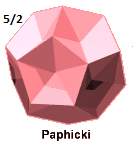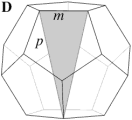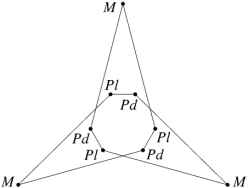Acronym dudsi Name dual of D-stellation of icosahedron,D-faceting of dodecahedron,vertex figure of paphicki©© Circumradius sqrt(3)/2 = 0.866025 Vertex figure© Coordinates (1/2, 1/2, 1/2)   & all permutations, all changes of sign (vertex inscribed cube) (τ/2, τ-1/2, 0)   & even permutations, all changes of sign where τ = (1+sqrt(5))/2 General of army v-doe

Although dudsi is more directly been seen as a faceting of the dodecahedron, it well can be considered dually as being related to a stellation of the icosahedron. For the latter the du Val namings of complete shells, being used in turn within the set of "the fifty-nine [stellated] icosahedra", got known as a standard. The former lack such a standard. This is why the acronym is based on the dual figure.

As an abstract polyhedron dudsi is isomorph to hudsi, thereby maintaining the q-edges, but replacing the v-edges by f-edges, resp. replacing the triangular faces from being stip vertex figures into being pip vertex figures. – It then still remains vertex inscribed into a v-doe.

Incidence matrix

20 |  6  3 |  9
---+-------+---
2 | 60  * |  2  q
2 |  * 30 |  2  v
---+-------+---
3 |  2  1 | 60  verf(stip)